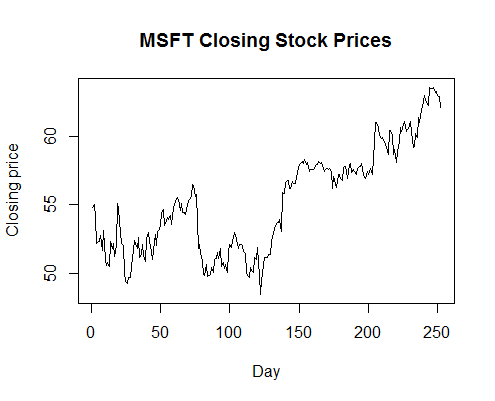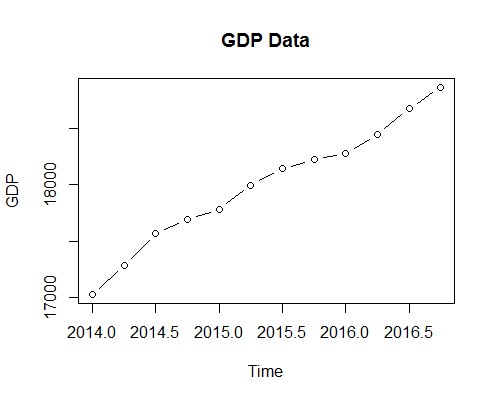# Plotting Time Series in R

While we can explore time series data using commands such as print()head()tail(), etc in R, it can be very helpful to plot the time series data as a line chart and explore it visually.

In the following examples, we plot the Microsoft stock data and the quarterly GDP data in two different plots using the plot() function in R. You can alternatively also use ts.plot() function.

> plot(msft_ts,xlab="Day",ylab="Closing price", main="MSFT Closing Stock Prices")> plot(GDP_data,xlab="Time",ylab="GDP", main="GDP Data",type="b")Both the series show an upward trend over time. The trend for the MSFT stock is not very clear during the first half of the year, however during the send half, it is a clear uptrend. The GDP graph on hand, has an uptrend since the beginning.

Get our Data Science for Finance Bundle for just $29$51.